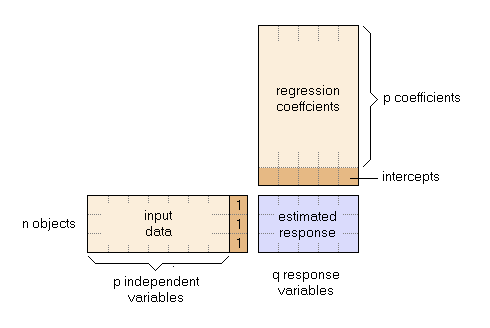The SDL Component Suite is an industry leading collection of components supporting scientific and engineering computing. Please visit the SDL Web site for more information....## RegCoeffUnit: SDL_statis Class: TPLSModel Declaration: property RegCoeff: TMatrix;

The readonly array property RegCoeff contains the regression coefficients of the PLS model. Each column of the RegCoeff matrix contains the regression coefficients for a particular output variable. The first p rows contain the regression coefficients for the p input variables, the intercept of the model is stored in the last row of the matrix.

In order to calculate the reponses for the input data, you have to augment the input matrix by an extra column containing all 1.0. In this way the intercepts are automatically included when multiplying the two matrices.Hint: A more convenient way to calculate the reponses is to use the method Predict.

Last Update: 2012-Oct-20